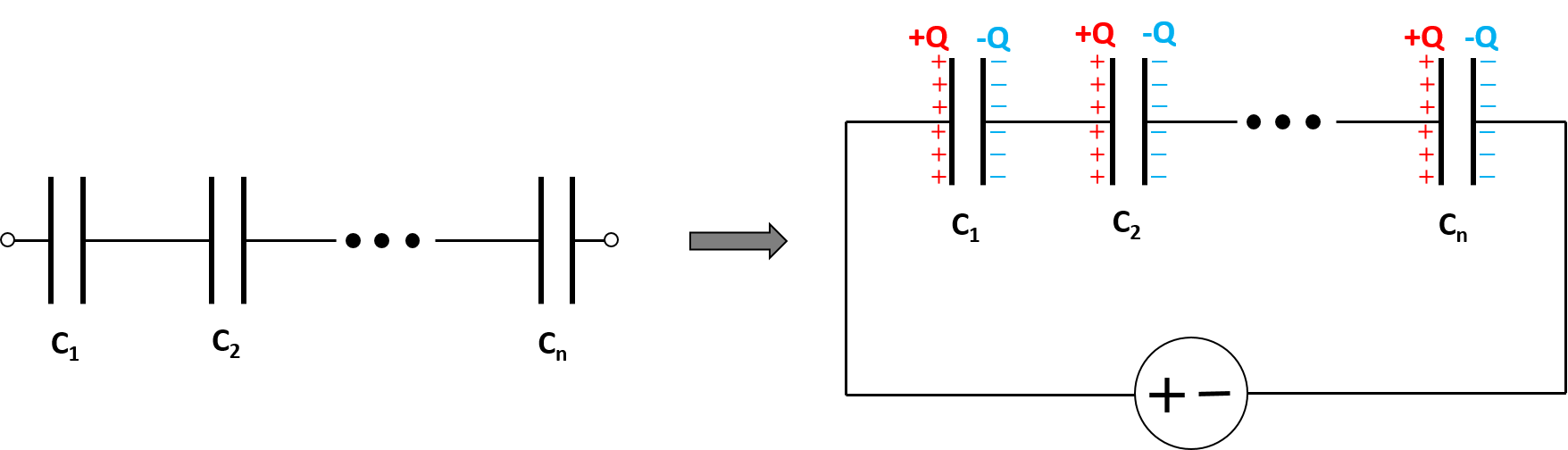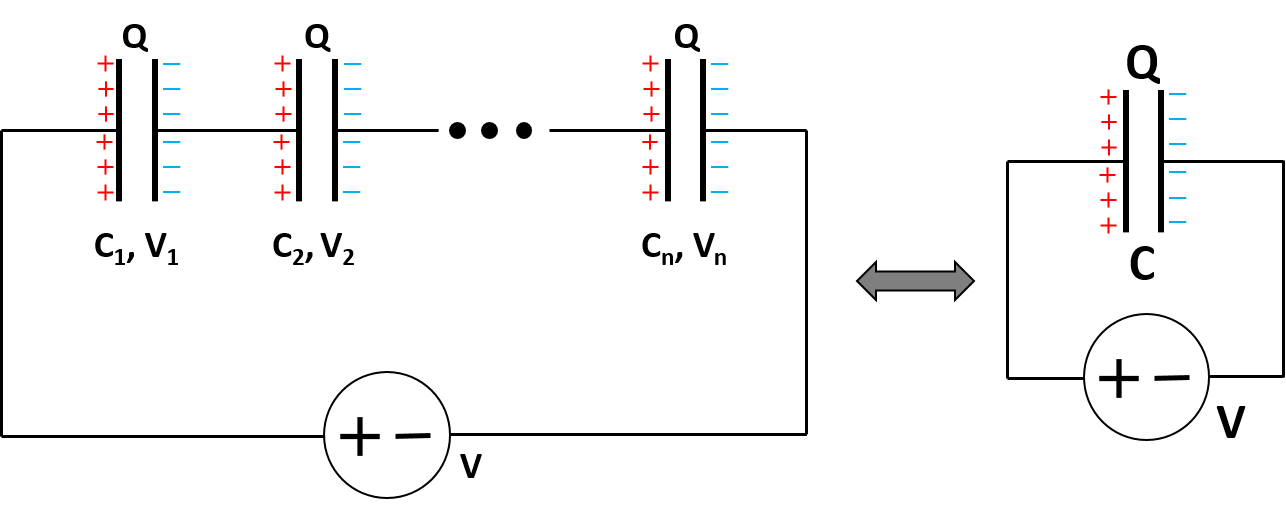# Capacitors in Series Calculator

Created by Wojciech Sas, PhD candidate
Reviewed by Bogna Szyk and Jack Bowater
Last updated: Nov 02, 2021

This capacitors in series calculator helps you evaluate the equivalent value of capacitance of up to 10 individual capacitors. In the text, you'll find how adding capacitors in series works, what the difference between capacitors in series and in parallel is, and how it corresponds to the combination of resistors. If you want to familiarize yourself with the formulas (and physics!) of this phenomena, check the example - you can work out capacitor in series voltage and charge.

## Adding capacitors in series

A capacitor is an electronic component where electric charge (or electrical energy) is stored. Capacitors can be arranged in a circuit, both in series and parallel, depending on their future application. When they are combined in series, the charge, Q, in each capacitor is the same. Why?

Imagine a setup composed of capacitors in series but without any source of voltage. The simplest way to visualize this situation is by using parallel plate capacitors, but it also works for cylindrical and spherical ones. In our case, each of the elements stores no charge. We then apply a voltage at the ends of the circuit.Look at the first capacitor - as electrons move to the power source, one part of the capacitor becomes positively charged. In equilibrium, this value is +Q. The fundamental property of a capacitors is that the absolute value of the charge stored on both plates is the same but of opposite signs. As a result, the second end of this element has a charge of -Q. Next, this charge can't be produced out of nothing, so it must come from the second capacitor, and that's why it also stores a charge of +Q on the adjacent end. We can continue this reasoning over and over until we reach the last element. Here, the charge doesn't come from the neighboring capacitor, but from the voltage source instead.

You can think about this problem as it was a black box. Charge leaves the power source from one end, goes through the box, and comes back from the other side. The overall charge has to be conserved, so the input and output values must be equivalent.

On the other hand, the voltage of capacitors in series, V, is the sum of voltages over each one separately (V₁, V₂, ...). Rearranging the general formula for capacitance, we obtain the expression for the voltage over the whole circuit:V = Q / C,

as well as for each one individually:

V₁ = Q / C₁, V₂ = Q / C₂, etc.

Once again, adding capacitors in series means summing up voltages, so:

V = V₁ + V₂ + ...Q / C = Q / C₁ + Q / C₂ + ....

We can divide each side by Q, and then we get the final form of the capacitance formula (or its inverse, precisely speaking):

1 / C = 1 / C₁ + 1 / C₂ + ....

In other words, the inverse of total capacity is the sum of the inverses of every single capacitance.

## How to use capacitors in series calculator?

Let's take a look at a computational example. What is the total capacity of four capacitors in series, where the capacitance for each one is C₁ = 2 mF, C₂ = 5 µF, C₃ = 6 µF, C₄ = 200 nF?

1. Convert the units so that they are all the same. We can use scientific notation to make things simpler. By putting everything in terms of F, we get: C₁ = 2·10⁻³ F, C₂ = 5·10⁻⁶ F, C₃ = 6·10⁻⁶ F, C₄ = 2·10⁻⁷ F

2. Add the inverses of capacitance: 1/C = 1 / (2·10⁻³ F) + 1 / (5·10⁻⁶ F) + 1 / (6·10⁻⁶ F) + 1 / (2·10⁻⁷ F) = 5.367·10⁶ 1/F

3. Evaluate the inverse of this sum: C = 1 / (5.367·10⁶ 1/F) = 1.863·10⁻⁷ F

4. We can write the final result for adding capacitors in series using the appropriate prefix: C = 186.3 nF

Note that this outcome may be less precise than the one from the capacitors in series calculator, because we don't use as many significant figures here.

If you look closer, you will notice that the resulting capacitance is lower than any of the individual values for capacitors in series.

## Capacitors in series and in parallel

As we've already found out, the arrangement of capacitors in series results in a capacitance of a lower value. As you may expect, combining capacitors in parallel increases the value. We can also see some similarities between different types of electric elements:

RC circuits consist of a combination of resistors and capacitors. Sometimes, there is more than one of each element, but it's usually possible to turn each of them into a single component. If the capacitors are arranged in parallel, you'll know how to estimate the outcome!

Some voltage dividers are built of capacitors in series. Check out our calculator to find some of their benefits are over standard resistive dividers!

The 555 IC, in its astable mode, uses two capacitor in series to define its characteristic operation times. If you need a timer in your circuit, try our 555 timer calculator.

Wojciech Sas, PhD candidate
You can arrange up to 10 capacitors
Capacitor 1 (C₁)
F
Capacitor 2 (C₂)
F
People also viewed…

### Chilled drink

With the chilled drink calculator you can quickly check how long you need to keep your drink in the fridge or another cold place to have it at its optimal temperature. You can follow how the temperature changes with time with our interactive graph.

### Gravitational time dilation

With this gravitational time dilation calculator you can check how much time slows down by near big objects.

### Porosity and permeability

The porosity and permeability calculator uses Darcy's law to calculate these two material properties as fluids flow through porous substances.

### Social Media Time Alternatives

Check what you could have accomplished if you get out of your social media bubble.